# Vector Basics: Describing Directions

We’re looking at the concept of vectors at an introductory level. Last week we looked at how they are defined in this context (as quantities with magnitude and direction), and how they are added (which is really part of the definition). Our collection of answers from Ask Dr. Math this time focuses on the ideas of unit vectors, components, and “direction cosines”, which are all ways to describe the direction of a vector.

## Unit vectors and collinearity

The first question is from 2002:

Unit Vectors

I am trying to solve a math problem that I truly do not understand.  The problem reads:
"Find the two unit vectors that are collinear with each of the following vectors.  (a) vector A = (3, -5)"

That's the first question in this problem, anyway.

I don't understand what this problem is even asking me to do.  Is a unit vector only ever equal to 1?  I've done a lot of research in my book and on the internet and I still don't understand.  Any help you could provide would be GREATLY appreciated.

Thanks ever so much.

A unit vector is one whose length (magnitude) is 1; collinear vectors lie along the same line (so they can go in the same or opposite directions). Doctor Ian answered:

A unit vector can have any direction, but its length is equal to 1.  So the following are all unit vectors:

(0,1)               length^2 = 0^2 + 1^2 = 1

(1,0)               length^2 = 1^2 + 0^2 = 1

(1/2, sqrt(3)/2)    length^2 = (1/2)^2 + (sqrt(3)/2)^2 = 1



The length, or magnitude, of a vector is found by the Pythagorean theorem: $$|(a,b)| = \sqrt{a^2+b^2}$$

Here are his three vectors, showing that they all have length 1: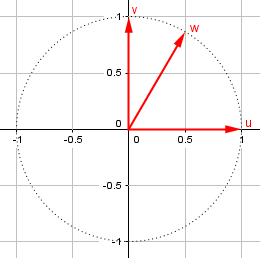In fact, if you pick any point on the unit circle (i.e., the
circle centered at the origin, whose radius is 1), the vector
from the origin to the point is the unit vector (cos(a),sin(a)),
where a is the angle from the positive x-axis to the point.

Here is an example, where my angle $$\theta$$ is 133°, so its components are $$(\cos(133°), \sin(133°)) = (-0.68, 0.73)$$: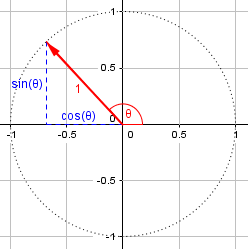The easiest way to get a unit vector that is collinear with a vector (a,b) is to find the magnitude of the vector,

|(a,b)| = sqrt(a^2 + b^2)

and divide both components by that:

1/|(a,b)| * (a,b) = (a/|(a,b)|, b/|(a,b)|)

Do you see why this will always be collinear with the original vector, and why its length will always be equal to 1?

(Note that the unit vector that points in the _opposite_ direction is also collinear.)

Dividing both components by $$|a|$$ reduces the length to 1 without changing the direction. Multiplying by negative 1 reverses the direction. Here are our vector $$\mathbf{a} = (3, -5)$$and the two unit vectors $$\mathbf{u}_a = \left(\frac{3}{\sqrt{34}}, -\frac{5}{\sqrt{34}}\right)$$ and $$-\mathbf{u}_a = \left(-\frac{3}{\sqrt{34}}, \frac{5}{\sqrt{34}}\right)$$: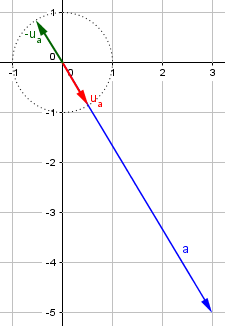## Unit vectors, basis vectors

This 1998 question is from a student whose goals are far beyond the basics, but who needs help starting:

Unit and Basis Vectors in Three Dimensions

Please give me a simple explanation of:

1. Unit vector.

My books (e.g., _Vector and Tensor Analysis_ by Borisenko) are not clear and assume I already understand this. Also, what use is a unit vector?

2. Basis vector. Again, my other sources are not clear.

P.S. I study relativity on my own, and this is why I'd like to understand the basics, like tensor algebra.

Doctor Anthony answered, giving the basic definition for vectors that we discussed last time, because it is applicable (with some little modifications) to physics:

A vector is a physical quantity, like velocity, displacement, or force, having both magnitude and direction.

Think of a vector as represented by a straight line pointing in a particular direction. The length of the line represents the magnitude of the vector. So in the case of a unit vector, the length of the line is 1 unit.

It is convenient to use unit vectors when working on problems. If we let u represent a vector in a certain direction and of unit magnitude, then 3u, 7u, and -8u are immediately understandable as vectors of magnitudes 3, 7 and -8 all in the direction of u (except -8u, as the negative sign means "in the opposite direction to +u").

Just as, above, we started with a vector a and found a unit vector in the same direction, here we can reverse the process and describe a vector as a unit vector in the right direction, multiplied by its length. In doing this, we are splitting the vector into its magnitude (a number) and its direction (a unit vector). Here are a unit vector u and the multiples that were mentioned: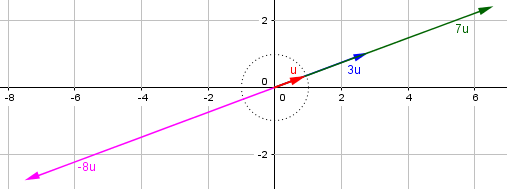But we can do much more by picking a standard set of unit vectors to use as a “basis” for describing any vector at all:

It is very common to use i, j, and k as unit vectors in the directions of the x, y, and z axes, respectively, in 3D space. This means that EVERY vector in space can be given in terms of its "components" parallel to those three axes. So, for example, 5i + 2j - 6k is a vector in space, and its magnitude would be represented by the length of a line joining the origin (0,0,0) to the point (5,2,-6).
Incidentally, this answers your second question: i, j, k are called "base" vectors because they are used as the basis for expressing all other vectors. Every other vector in 3D space whatsoever can be given in terms of i, j, and k.

I’ll stick to two dimensions here. The unit basis vectors i and j are the same as u and v that we used in the first illustration above: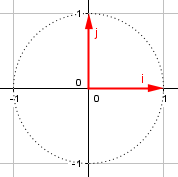Sometimes it is convenient to use other vectors as base vectors. Any two non-parallel vectors could be used as base vectors to give any point in the plane of the two vectors. That is, every other vector in that plane could be expressed in terms of the base vectors, just as we say 6i + 4j to express a vector in the xy plane. Similarly, any three non-coplanar vectors could be used as base vectors "spanning" 3D space. Again, the most common base vectors are i,j, k, but there are occasions when an entirely different set of base vectors are used.

Finally, vectors are not confined to 1, 2, or 3 dimensions. You can
have multi-dimensional vectors expressed in terms of 4, 5, 6, and
higher base vectors. The number of base vectors will equal the
dimension of the space under consideration.

Here is our vector 6i + 4j, which can also be called (6, 4) using its components: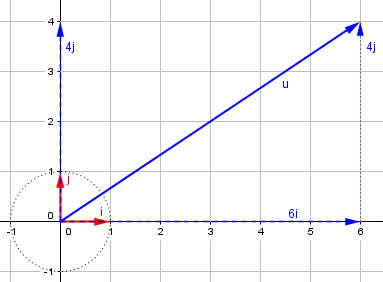## Direction as angles (2 dimensions)

The next question, from 1998, involves vectors whose direction is expressed as the angle from the positive x-axis:

Vector Components, Magnitude, and Direction

Vector M of magnitude 4.75 m is at 58.0 degrees counter-clockwise from the positive x-axis. It is added to vector N, and the resultant is a vector of magnitude 4.75 m, at 39 degrees counterclockwise from the positive x-axis. Find: (a) the components of N, and (b) the magnitude and direction of N.

I drew a graphical illustration of the problem. But I really can't solve it because I don't know how.

Here we have two vectors being added, and one of them and the sum are described in terms of magnitude and direction. We want to find vector n, both in terms of components and of direction (angle) and magnitude: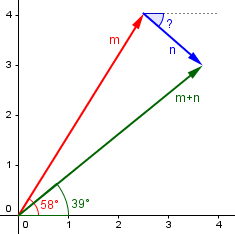Doctor Rick answered, suggesting the most likely method:

Hi, Kristine,

I will get you started on solving this kind of problem. There are two tools you need to do this: (1) converting between magnitude/direction and components of a vector and (2) adding vectors. The first requires some trigonometry, so I hope you've had some.

(1) You are given the magnitude and direction of vectors M and P (the sum of M and N). Before you can add them, you must find their components. Remember this diagram:

My+-------------* M
|            /|
|           / |
|          /  |
|         /   |
|        /    |
|      L/     |
|      /      |
sin(a)|-----+       |
|    /|       |
|  1/ |       |
|  /  |       |
| /)a |       |
|/____|_______|__________
O    cos(a)    Mx

A vector of length 1 has components (cos(a), sin(a)). By similar triangles, a vector M of length L has components Mx = L*cos(a), My = L*sin(a). Do this with both vectors M and P to get their components (Mx, My) and (Px, Py).

As we saw above, we can think of the vector m as a unit vector in the given direction multiplied by its length. The trigonometric functions cosine and sine give the x and y components of the unit vector, as we saw in our first answer. For our vector m, the angle is 58° and the length is 4.75, so the vector is $$(m_x, m_y) = (4.75\cos(58°), 4.75\sin(58°)) = (2.517, 4.028)$$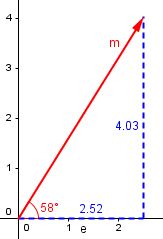Similarly, for vector p = m + n, we have angle 39° and length 4.75, so the vector is $$(p_x, p_y) = (4.75\cos(39°), 4.75\sin(39°)) = (3.691, 2.989)$$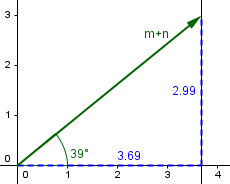(2) You know that M + N = P. To add vectors, add their components:

Mx + Nx = Px
My + Ny = Py

You know Mx, My, Px, and Py, so you should be able to figure out Nx and Ny. These are the components of vector N.


To find the components of n, we just subtract: $$n = (n_x, n_y) = (p_x-m_x, p_y-m_y) = (3.691 – 2.517, 2.989 – 4.028) = (1.174, -1.039)$$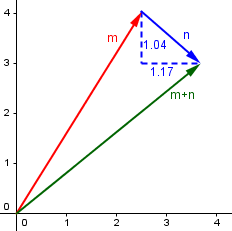That’s the answer to part (a).

(3) You were also asked for the magnitude and direction of vector N. To do this, you have to reverse step 1. Here's how, using the figure (remember, you'll be doing this for vector N, not vector M).

Magnitude(M) = Mx^2 + My^2
(the Pythagorean Theorem where ^2 means square)

tangent(a) = sin(a)/cos(a) = My/Mx (by similar triangles again)

So Direction(M) = a = inverse tangent of a = arctan(a)

The Pythagorean theorem gives our length as $$|\mathbf{n}| = \sqrt{n_x^2+n_y^2} = \sqrt{1.174^2+(-1.039)^2} = \sqrt{2.457797} = 1.568$$

The tangent of our angle is the slope of the vector: $$\tan(\theta) = \frac{n_y}{n_x} = \frac{-1.039}{1.174} = -0.885$$

So the angle itself is $$\theta = \tan^{-1}(-0.885) = -41.5°$$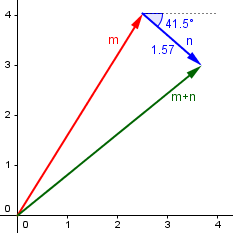Those are the tools you'll need. See if you can do the job now. Write back if you're still confused after you've tried it.

Presumably, Kristine did just what we’ve done here.

## Direction of a line (3 dimensions)

This 1997 question hopes for a way to indicate the direction of a 3-dimensional vector similar to the angle or slope in the previous type of problem:

Formula for Slope of 3-D Line

Thank you for answering our question about finding the length of a line in three dimensions.  Now we would like to know the formula to find the slope of a three-dimensional line.  We searched through textbooks and tried to adapt the formula, but with no success.

There is no direct analogue of the idea of slope in two dimensions. The subject you are discussing is analytic geometry of three dimensions. The following facts should help a little.

A linear equation in x, y, and z, such as ax + by + cz = d, is the equation of a plane, not a line.  Such equations can be put into one standard form by dividing by sqrt(a^2+b^2+c^2).  The resulting coefficients of x, y, and z have the property that the sum of their squares is 1.  Another standard form is gotten by dividing by d, and writing the equation as x/(d/a) + y/(d/b) + z/(d/c) = 1.  From this form you can read off the intercepts with the x-, y-, and z-axes: (d/a, 0, 0) is the x-intercept, (0, d/b, 0) is the y-intercept, and (0, 0, d/c) is the z-intercept (provided all of a, b, c, and d are nonzero).  One way of regarding the "slope" of a plane is to write down a unit vector which is perpendicular to it, called the normal vector.  It is given by (a*I + b*J + c*K)/sqrt(a^2+b^2+c^2), where I, J, and K are the unit vectors in the x, y, and z directions.  The coefficients of I, J, and K in this expression are called the direction cosines of the vector, because they are the cosines of the angles between the vector and the x-, y-, and z-axes, respectively.

The two standard forms he mentions for a plane are, in effect, $$a’x + b’y + c’z = d’$$ where the vector (a‘, b‘, c‘) is a unit vector called the unit normal vector (this is something we will see later in this series or a subsequent series), and $$\frac{x}{A} + \frac{y}{B} + \frac{z}{C} = 1$$ where A, B, and C are the intercepts on the three axes. The normal vector represents the direction of the plane.

But the question was about a line, and the “direction cosines” just mentioned for the unit normal vector show up here, too:

A line is specified as the intersection of two nonparallel planes. This means you need two linear equations in x, y, and z to determine a line.  There are several standard forms for the equations of a line, but a commonly used one is

x - x0   y - y0   z - z0
------ = ------ = ------
a        b        c

Here (x0, y0, z0) is a point on the line, and the numbers a, b, and c determine the direction along the line: the vector a*I = b*J + c*K is parallel to the line.  (Note: This form only works when the line is not parallel to any of the xy-, xz-, or yz-planes, i.e., when neither a, b, or c is zero).

Note that this form is not a single equation, but a pair of equations that set three quantities equal. In this form, $$\frac{x – x_0}{a} = \frac{y – y_0}{b} = \frac{z – z_0}{c}$$ the vector (a, b, c) gives the direction of the line, which is the best answer to the question, much like the normal vector for the plane.

In some sense, the direction cosines are the closest analogue to the slope.  In two dimensions, they are just the cosine of the inclination, which is the angle with the x-axis, and the cosine of its complement, which is the sine of the inclination. The slope is the ratio of these two, the tangent of the inclination. There is no exact analogue because there is no "ratio" of three direction cosines, or of any three numbers.

We could say, however, that the triple ratio a : b : c is a reasonable analogue of the slope of a line, even though it is not a number; the direction cosines, as we’ll see below, are just the components of the unit vector in the direction of the line.

As he suggests, we can do all this in two dimensions for comparison, which is quite instructive. We can write a line as $$\frac{x – x_0}{a} = \frac{y – y_0}{b}$$ which (solving for y) can be rewritten as $$y = \frac{b}{a}(x – x_0) + y_0$$ The slope is the number $$\displaystyle\frac{b}{a} = \frac{\cos(\theta_y)}{\cos(\theta_x)} = \frac{\sin(\theta_x)}{\cos(\theta_x)} = \tan(\theta)$$, showing that the ratio a : b is closely related to the slope, which is the tangent of the angle to the x-axis.

## Direction cosines of a vector

For a fuller picture of direction cosines, we’ll close with this question from 2003:

Why They're Called Direction Cosines

I would like to know how to find the angles between a 3D vector and the 3 coordinate axes, given the components of the vector.

Doctor Ian answered, using a concept we’ll be getting to next week, the dot product:

Hi Kristen,

If you have two vectors, A and B, and you want to find the angle between them, one way is to use the dot product:

dot(A,B) = |A||B|cos(theta)

Does that look familiar?  To find the angle between a vector and a particular axis, you can just make B a unit vector.  For example, if A is (a,b,c), then to find the angle with the x-axis,

_______________
a*1 + b*0 + c*0 = \|a^2 + b^2 + c^2 cos(theta)

a
-------------------- = cos(theta)
_______________
\|a^2 + b^2 + c^2

What he’s done here is to apply his formula for the angle between two vectors to the given vector a and the unit vector $$\mathbf{i} = (1,0,0)$$. The general formula for the angle between vectors a and b is $$\cos(\theta) = \frac{\mathbf{a}\cdot\mathbf{b}}{|\mathbf{a}||\mathbf{b}|}$$ and if we replace b with a unit vector u (either i, j, or k), we have $$\cos(\theta) = \frac{\mathbf{a}\cdot\mathbf{u}}{|\mathbf{a}|}$$ where the numerator is just the appropriate component of a.

So the cosine of the angle between a vector and the x-axis is just the x-component of the vector divided by the magnitude of the vector. This is true for each of the components. But you may recognize that this cosine is simply a component of the unit vector:

Note that if you make A a unit vector (which you can do by dividing all the components by the magnitude of A), you end up with

a    b    c
( ---, ---, --- ) = ( cos(theta ), cos(theta ), cos(theta ) )
|A|  |A|  |A|                x            y            z

For this reason, the components of a unit vector are often called the 'direction cosines' of the vector.

Does this help?

So for example, if we have the vector (3, 4, 5), whose magnitude is $$\sqrt{3^2 + 4^2 + 5^2} = \sqrt{50} \approx 7.07$$, then the unit vector in the same direction is $$\left(\frac{3}{\sqrt{50}}, \frac{4}{\sqrt{50}}, \frac{5}{\sqrt{50}}\right) = (0.424, 0.566, 0.707)$$ so the angles with the axes are the inverse cosines of these numbers, 64.9°, 55.6°, and 45°, respectively: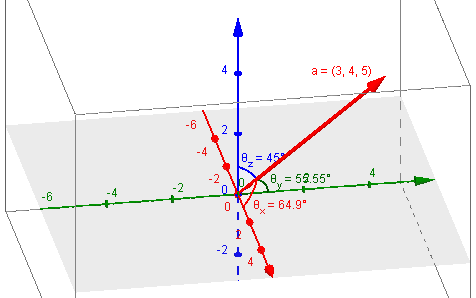Kristen replied,

Thank you for your help. That will make my life SO much easier!

Next week, we’ll look at ways to multiply two vectors.

### 1 thought on “Vector Basics: Describing Directions”

This site uses Akismet to reduce spam. Learn how your comment data is processed.